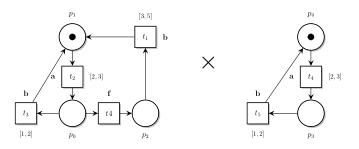# Diagnosability of TPN

One possible application of Twina, and our initial motivation for this work, is to check the diagnosability of a TPN. We consider a system with a distinguished event that models an unobservable fault (say all transitions with the label $f$). Basically, a system is diagnosable if, when looking only at the observable events and at their timing, we can always detect when an execution contains a fault; and if so after a finite number of observations.

A simple method to check the diagnosability of a TPN is to adapt the Twin Plant method [SHCK01]. The idea is to build a diagnoser, that is the intersection of two copies of the same system: one with the fault, $N.1$, and another without it, $N.2$, and to check whether we can find an infinite execution with a fault in their intersection. Indeed, if this is the case, then there is an infinite execution after a fault in $N.1$ that can be mistaken with a non-faulty one (in $N.2$). We give an example of this construct in Fig. 1.The twin product for TPN faulted.net and fault $f$

In this system, it is not enough to look at the sequence of events: even after a fault, we always observe a $b$ after an $a$. Nonetheless, in the product, every execution where transition $t_4$ fires leads to a time deadlock. Indeed, in this case, we must wait at least 3 (time units) to fire transitions $t_1$ and at most 2 to fire $t_5$ (and both have label $b$). This means that the system is diagnosable. Actually, we detect the occurrence of a fault whenever $b$ does not appear at most 2 (time units) after an $a$.

The twin-plant construction is quite useful and we provide an option to directly build a twin TPN in our tool (with option -twin).

$twina -fault=f -twin -v -lt faulted.net # states 3 # transitions 3 class 0 marking: {p1.1} {p1.2} domain: 2 <= {t2.1} <= 3 2 <= {t2.2} <= 3 successors: {t2.1}|{t2.2}:"a"/1 class 1 marking: {p0.1} {p0.2} domain: 1 <= {t3.1} <= 2 0 <= {t4.1} < w 1 <= {t3.2} <= 2 successors: {t3.1}|{t3.2}:"b"/0 {t4.1}:"f"/2 class 2 marking: {p2.1} {p0.2} domain: 3 <= {t1.1} <= 5 0 <= {t3.2} <= 2 successors:  In this case, we can generate a LTS for the twin plant and check that every fault eventually leads to a deadlock. For instance using a LTL model-checker and a property such as$(\lozenge f) \Rightarrow (\lozenge \mathrm{deadlock})$. In the example below we show how to use option -kaut of Twina, in association with option -tina of Ktzio, to generate an output in the KTZ format preserving the labels of the net (option -lt). Then we can use this file with selt, the LTL model-checker provided with Tina. $ twina -fault=f -twin -kaut -lt faulted.net | ktzio -tina -AUT - faulted.ktz
$selt -q faulted.ktz -f "(<> f) => (<> dead)" TRUE  We also provide a dedicated algorithm, option -diag of Twina, to check this property on-the-fly. When the system is not diagnosable, it allows us to find a counter-example before exploring the whole behavior of the twin-plant. Below, we confirm that the net faulted.net is diagnosable; but example basileTAC.net is not. Indeed we find a loop of 6 transitions in the intersection between the net and its twin. $ twina -fault=f -diag faulted.net

# net is diagnosable
#
# states (explored):   3
# markings:            3
# dbms:                3

\$ twina -fault=f -diag -v -lt basileTAC.net
# net is not diagnosable
# length: 6
(*) class 0
marking: {p0.1} {p0.2}
domain:
2 <= {t4.1} <= 2
1 <= {t5.1} <= 2
2 <= {t4.2} <= 2
1 <= {t5.2} <= 2
----[{t4.1}:"i"]--------->
class 1
marking: {p1.1} {p0.2}
domain:
1 <= {t1.1} <= 2
0 <= {t4.2} <= 0
0 <= {t5.2} <= 0
----[{t4.2}:"i"]--------->
class 2
marking: {p1.1} {p1.2}
domain:
1 <= {t1.1} <= 2
1 <= {t1.2} <= 2
----[{t1.1}|{t1.2}:"a" (*)]->
class 3
marking: {p2.1} {p2.2}
domain:
1 <= {t2.1} <= 2
0 <= {t6.1} <= 2
1 <= {t2.2} <= 2
0 <= {t6.2} <= 2
----[{t6.1}:"i"]--------->
class 4
marking: {p3.1} {p2.2}
domain:
1 <= {t7.1} <= 1
0 <= {t2.2} <= 2
0 <= {t6.2} <= 2
{t6.2} - {t2.2} <= 1
----[{t7.1}:"f"]--------->
class 5
marking: {p4.1} {p2.2}
domain:
1 <= {t3.1} <= 2
0 <= {t2.2} <= 1
0 <= {t6.2} <= 1
----[{t3.1}|{t2.2}:"b" (*)]->
class 0



## Examples used in this post

A basic examples of net with fault from our paper faulted.net

An example from [Basile2015]: basileTAC.net .